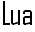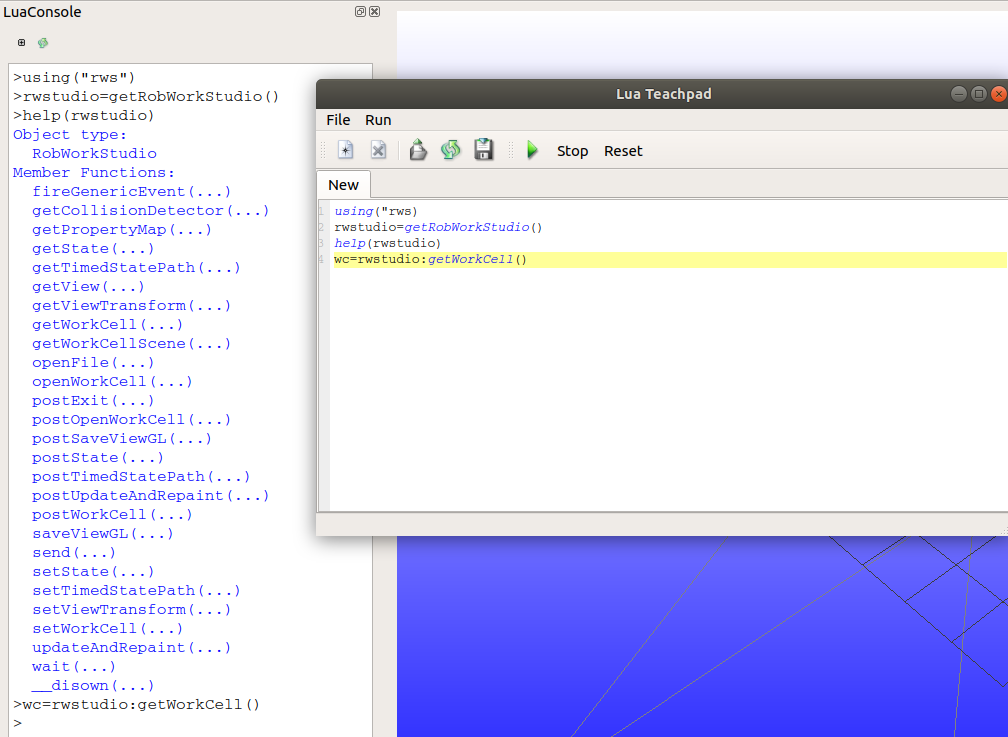# Lua Interface¶

RobWork has an interface to the Lua scripting language. This section will present examples and general use patterns for using Lua in RobWork.

In general most functionality in the Lua interface is generated using SWIG. There is no automatic generation of API documentation for the Lua interface, but instead it is encouraged that you use the C++ API Reference, Java API Reference, or Python API Reference. Notice that it is also possible to call the help(object) function in Lua to print a list of available functions on a given object.

Lua can either be used as a stand-alone script in a generic interpreter, in a RobWork-specific interpreter or inside the RobWorkStudio Lua plugin. There are slight differences in how the scripts should be written and how they are run, which will be covered in the following sections.

## Stand-alone Scripts¶

To run the script with a standard Lua interpreter, it is necessary to setup the LUA_CPATH environment variable to let the interpreter know where to find the RobWork Lua modules.

In Linux, this could for instance be set with the following command:

export LUA_CPATH="/path/to/RobWork/libs/BUILD_CONFIGURATION/lib?_lua.so;/path/to/RobWorkStudio/libs/BUILD_CONFIGURATION/?_lua.so;/path/to/RobWorkSim/libs/BUILD_CONFIGURATION/?_lua.so"


Adjust the paths to actual path names on the system.

When running the Lua script, the modules must be loaded using the require function:

require("rw")
require("rw_simulation")


## Building a Lua interpreter¶

It is possible to compile a Lua interpreter that has the RobWork types built in directly. The rwlibs::swig::openLuaLibXX functions are used for this, where XX is something different for each Lua module.

This program loads all the RobWork Lua modules into a Lua interpreter and runs a Lua script given on the command line:

  1 2 3 4 5 6 7 8 9 10 11 12 13 14 15 16 17 18 19 20 21 22 23 24 25 26 27 28 29 30 31 32 33 34 35 #include #include #include #include #include #include #include #include #include int main(int argc, char** argv) { if (argc != 2) { std::cerr << "Usage: " << argv << " \n"; return 1; } lua_State *L = luaL_newstate(); luaL_openlibs(L); rwlibs::swig::openLuaLibRW_rw(L); rwlibs::swig::openLuaLibRW_rw_assembly(L); rwlibs::swig::openLuaLibRW_rw_control(L); rwlibs::swig::openLuaLibRW_rw_pathoptimization(L); rwlibs::swig::openLuaLibRW_rw_pathplanners(L); rwlibs::swig::openLuaLibRW_rw_proximitystrategies(L); rwlibs::swig::openLuaLibRW_rw_simulation(L); rwlibs::swig::openLuaLibRW_rw_task(L); const int error = luaL_dofile(L, argv); if (error) std::cerr << lua_tostring(L, -1) << "\n"; lua_close(L); return error; } 

RobWorkStudio and RobWorkSim provide similar functions.

Notice that this interpreter will not need the scripts to call require to load the RobWork modules. They are built in from the beginning.

## RobWorkStudio Lua¶

To see the LuaConsole plugin, open the Luaplugin in RobWorkStudio.

You will see the following LuaConsole plugin, and by clicking at the plus button in the top left corner, you get the Lua editor window “Lua Teachpad”.The LuaConsole plugin and the Lua editor window.

In the LuaConsole and Lua Teachpad, you will not need to use the require function to load RobWork Lua libraries. They are already available in the interpreter used internally by RobWorkStudio.

## Conventions & Utilities¶

The following conventions are used in the Lua interface:

• RobWork math types are templated with the precision used, such as Rotation3D<double> and Rotation3D<float>. In the script interfaces, this can not be mapped directly. Instead those types are mapped to the types Rotation3d and Rotation3f. For math types we follow this convention with d and f for double and float precision respectively.

• The types must be written with a prefix giving the name of the Lua module where it origins. For instance it is necessary to write rw.Q or rw_simulation.Simulator. The using utility function is provided to allow the user to import the types a global names, such as using(“rw”) and using(“rw_simulation”). This is somewhat similar to the “using namespace” statements in C++.

Example of the construction of a Q vector without and with the using function:

-- rw prefix is necessary to create Q:
q1 = rw.Q(2,{1,2})
-- Import the rw types to global namespace:
using("rw")
-- rw prefix can now be left out
q2 = Q(2,{1,2})


## Lua program example¶

This example showcases most of the functionality of the Lua script interface:

  1 2 3 4 5 6 7 8 9 10 11 12 13 14 15 16 17 18 19 20 21 22 23 24 25 26 27 28 29 30 31 32 33 34 35 36 37 38 39 40 41 42 43 44 45 46 47 48 49 50 51 52 53 54 55 56 57 58 59 60 61 62 63 64 65 66 67 68 69 70 71 72 73 74 75 76 77 78 79 80 81 82 83 84 function b2s(b) if b then return "true" else return "false" end end x = 2.92 print("Radians to degrees and back:", b2s(rw.d2r(rw.r2d(x)) == x)) print() print("rpy is", rw.rpy(0.3, 0, 0)) print("rpy is", rw.rpy_deg(rw.r2d(0.3), 0, 0)) print("eaa is", rw.eaa(0.3, 0, 0)) print("eaa is", rw.eaa_deg(rw.r2d(0.3), 0, 0)) print() q = rw.Q({1, 2, 3, 4, 5, 6, 7}) print("q is", q) print() v = rw.Vector3D({0, 3, -0.3}) print("v is", v) print("v * 2 is", v * 2) print("v + v - v is v:", b2s(v + v - v == v)) print() r = rw.Rotation3D({1, 0, 0, 0, 1, 0, 0, 0, 1}) print("r is", r) print("r^2 is", r * r) print("rw.inverse(r) is", rw.inverse(r)) print("r:inverse() is", r:inverse()) print("The type of Rotation3D is", tolua.type(r)) print() t = rw.Transform3D(v, r) print("t is", t) print("rw.inverse(t) is", rw.inverse(t)) print("t:inverse() is", t:inverse()) print("The rotation of t is", t:r()) print("The translation of t is v:", b2s(v == t:p())) print() print("r * v is", r * v) print("(t * t) * v is", (t * t) * v) print() workcell = rw.loadWorkCell("workcell.wu") print("Workcell is", workcell) state = workcell:getDefaultState() frame = workcell:getFrame("Device.TCP") print("Frame is", frame) world = workcell:getWorldFrame() print("World frame is", world) print("World to frame transform is", world:to(frame, state)) print("Frame world transform is", frame:wt(state)) print("Frame world translation is", frame:wt(state):p() - world:wt(state):p()) print() device = workcell:getDevice("Device") print("Device is", device) print("Device base is", device:getBase()) print("Device end is", device:getEnd()) print("Device configuration is", device:getQ(state)) print() q = rw.Q{ 0.98, 1.503, -1.521, -0.76, -0.237, -0.895, 0} print("Assigning device configuration to state.") device:setQ(q, state) print("Device configuration is assigned configuration:", b2s(device:getQ(state) == q)) print() item = workcell:getFrame("Item") pos = item:wt(state):p() print("Item position is", pos) rw.gripFrame(item, device:getEnd(), state) print("Item position is", item:wt(state):p()) item:attachFrame(world, state) print("Item position is", item:wt(state):p()) 

The output of running the script in the interpreter is as follows:

  1 2 3 4 5 6 7 8 9 10 11 12 13 14 15 16 17 18 19 20 21 22 23 24 25 26 27 28 29 30 31 32 33 34 35 36 37 38 39 40 41 42 43 44 45 46 Radians to degrees and back: true rpy is Rotation3D {0.955336, -0.29552, 0, 0.29552, 0.955336, 0, 0, 0, 1} rpy is Rotation3D {0.955336, -0.29552, 0, 0.29552, 0.955336, 0, 0, 0, 1} eaa is Rotation3D {1, 0, 0, 0, 0.955336, -0.29552, 0, 0.29552, 0.955336} eaa is Rotation3D {1, 0, 0, 0, 0.955336, -0.29552, 0, 0.29552, 0.955336} q is Q{1, 2, 3, 4, 5, 6, 7} v is Vector3D {0, 3, -0.3} v * 2 is Vector3D {0, 6, -0.6} v + v - v is v: true r is Rotation3D {1, 0, 0, 0, 1, 0, 0, 0, 1} r^2 is Rotation3D {1, 0, 0, 0, 1, 0, 0, 0, 1} rw.inverse(r) is Rotation3D {1, 0, 0, 0, 1, 0, 0, 0, 1} r:inverse() is Rotation3D {1, 0, 0, 0, 1, 0, 0, 0, 1} The type of Rotation3D is rwlibs::lua::internal::Rotation3D t is Transform3D(Vector3D {0, 3, -0.3}, Rotation3D {1, 0, 0, 0, 1, 0, 0, 0, 1}) rw.inverse(t) is Transform3D(Vector3D {0, -3, 0.3}, Rotation3D {1, 0, 0, 0, 1, 0, 0, 0, 1}) t:inverse() is Transform3D(Vector3D {0, -3, 0.3}, Rotation3D {1, 0, 0, 0, 1, 0, 0, 0, 1}) The rotation of t is Rotation3D {1, 0, 0, 0, 1, 0, 0, 0, 1} The translation of t is v: true r * v is Vector3D {0, 3, -0.3} (t * t) * v is Vector3D {0, 9, -0.9} Workcell is WorkCell[workcell.wu] Frame is Frame[Device.TCP] World frame is Frame[WORLD] World to frame transform is Transform3D(Vector3D {-0.955356, 0.107485, 0.161679}, Rotation3D {0.121395, -0.856918, -0.500954, -0.227824, 0.467159, -0.854318, 0.966105, 0.217839, -0.138515}) Frame world transform is Transform3D(Vector3D {-0.955356, 0.107485, 0.161679}, Rotation3D {0.121395, -0.856918, -0.500954, -0.227824, 0.467159, -0.854318, 0.966105, 0.217839, -0.138515}) Frame world translation is Vector3D {-0.955356, 0.107485, 0.161679} Device is Device[Device] Device base is Frame[Device] Device end is Frame[Device.J6] Device configuration is Q{0.98, 1.503, -1.521, -0.76, -0.237, -0.895, 0} Assigning device configuration to state. Device configuration is assigned configuration: true Item position is Vector3D {0, 0.5, 0.5125} Item position is Vector3D {1.07946e-016, 0.5, 0.5125} Item position is Vector3D {0.365482, -0.558872, -0.862516} 

## Executing Lua code in RobWorkStudio¶

Lua code can be executed in RobWorkStudio via the Lua plugin interface. The Lua plugin has some additional Lua functions in the c rw package to access variables of the RobWorkStudio environment.

c rw.getWorkCell() returns the currently loaded workcell:

w = rw.getWorkCell(); print(w)
--
WorkCell[D:/movebots/FanucSchunk/scene.wu]


c rw.getDevice(name) retrieves the device of the given name:

d = rw.getDevice("Robot"); print(d)
--
Device[Robot]


c rw.getState() returns a copy of the current workcell state of RobWorkStudio:

s = rw.getState(); print(d:getQ(s))
--
Q{0, 0, 0, 0, 0, 0}


The workcell state can be written back to RobWorkStudio with c rw.setState():

q = rw.Q{0.5, 0, 0, 0, 0, 0}; d:setQ(q, s); rw.setState(s)


This will update the position of the device as displayed in RobWorkStudio.

## Other Lua functions and methods¶

The interface has some additional experimental functions and methods that will be documented and expanded as RobWork matures.

device = rw.CompositeDevice(devices, state, options)
detector = rw.getCollisionDetector(options)
collision = detector:inCollision(state)
strategy = rw.getCollisionStrategy(options)
planner = rw.getPathPlanner(device,
{ tcp = frame, state = state, detector = detector })
path = planner:query(from, to)
path = rw.Path(states)
path1 + path2
rw.storeStatePath(workcell, path, file)
rw.getOutput()